Courses
Courses for Kids
Free study material
Free LIVE classes
More

# Knowledge of Maths for Class 3LIVE
Join Vedantu’s FREE Mastercalss

## An Introduction to Maths for Class 3

The third-grade Maths lessons are structured so that students can practise their Maths skills while engaging in third-grade Maths games. Every effort has been made to explain new concepts in simple language so that the kid may readily understand them, keeping in mind a child's mental ability in the third grade. The difficulty level of the exercises has been lowered, and mathematical ideas have been simplified to the greatest extent possible.

Each topic offers a substantial number of examples to understand how concepts are used. The key arithmetic topics we'll cover in third grade include four-digit numbers, number comparisons, addition, subtraction, multiplication, division, and geometric shapes and figures. The measurement of length, mass, capacity, time, money, fractional numbers, and pictographs.

## Formation of Four-digit Numbers

Four-digit numbers are those numbers that consist of only 4 digits in which the first digit should be 1 or greater than 1, and the rest of the digits can be any number between 0 and 9. For example, 5693, 1023, and 9825 are four-digit numbers.

4-digit numbers are written or read according to the place values of the digits. In 4-digit numbers, the four digits correspond to the four-place values - ones, tens, hundreds, and thousands. When a number is written in standard form, each group of digits is separated by a comma which forms a period.

For example, 3510 is a 4-digit number, and after placing the comma, it is written as 3,510.

## International and Roman Numerals

Roman numerals are created by converting numbers to their respective Roman digits. The numerals used in English or international numeration in the Hindu-Arabic system are 1, 2, 3, 4, 5, 6, 7, 8, 9, and 0. Roman numeral values for the international system are as follows:

One as I, Five as V, Ten as X, Fifty as L, Hundred as C, and Five hundred as D

Now, numbers to roman numerals as follows:

4 as IV, 9 as IX, 24 as XXIV, 20 as XX, 39 as XXXIX, 11 as XIInternational and Roman Numerals

## Comparing Numbers

We are going to show class 3 maths questions by using the topic of comparing numbers. In the end, we provide an image that shows numbers by comparing them.

• Step 1: To compare the digit count. The number increases as the number of digits increases.

• Step 2: Compare the higher place values if the number of digits is the same. For example, 789 and 889.

Here we can see that the ones and tens place values are the same, so in this case, we will compare the higher values, i.e. of hundred places. As 7 < 8. Therefore, 789 < 889.

• Step 3: Compare the digits in the next place value to the right if they are the same at the highest place value.

For Example, 789 and 779.

Here, out of 789 and 779, the hundred-place and ones place values are the same. So we will see the tens place value. As 8>7, therefore, 789>779.

• Step 4: Continue comparing digits with the same place value until you discover digits with a different place value. The greater number is the one with the higher face value.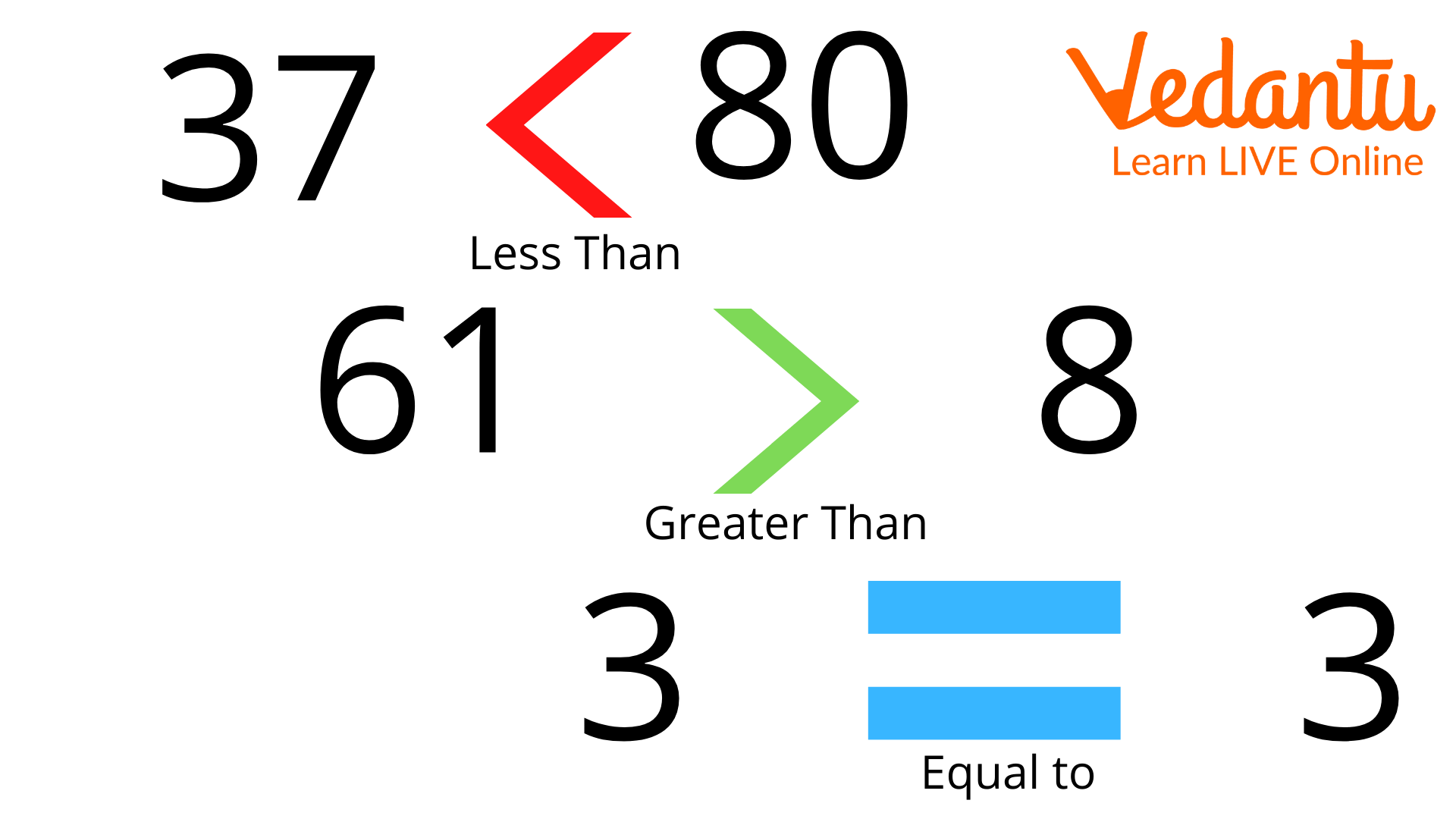Comparing Numbers

## Geometrical Shapes and Figures

In mental Maths for Class 3, we can show the concept of geometrical shapes and figures. Here, we also show examples of geometrical shapes in two- dimensional and in three- dimensional.

Geometric shapes are defined as figures closed by a boundary that combines a definite amount of curves, points, and line segments. Each shape has a unique name, such as a circle, square, triangle, rectangle, etc.

 2D Shapes 3D Shapes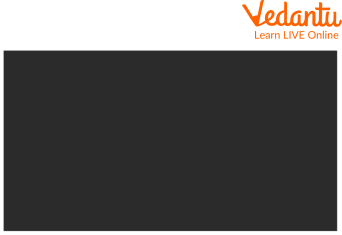Rectangle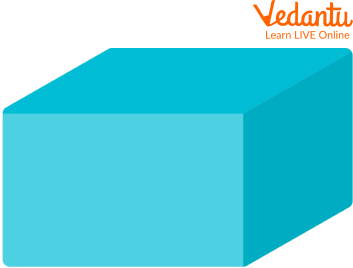Cuboid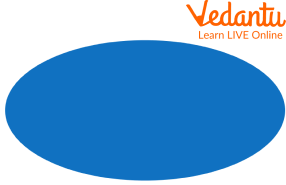OvalCylinder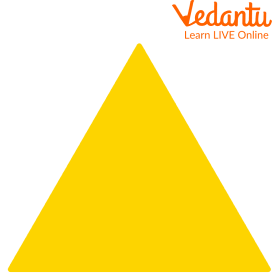Triangle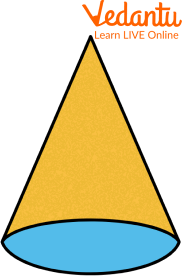Cone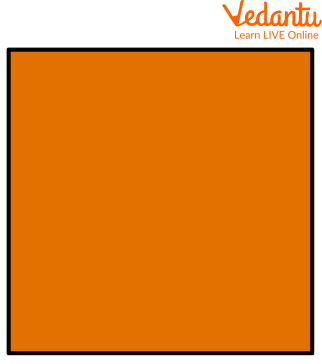Square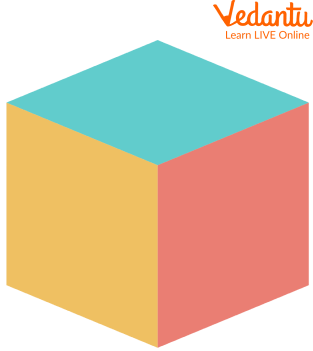Cube

## Class 3 Maths Chapter 1 Numbers

A number is a basic component of Mathematics. Numbers are used for counting, measuring, keeping things in order, indexing, etc. We have different types of numbers based on their properties such as natural numbers, whole numbers, rational and irrational numbers, integers, real numbers, complex numbers, even and odd numbers, etc in class 3 maths chapter 1 numbers.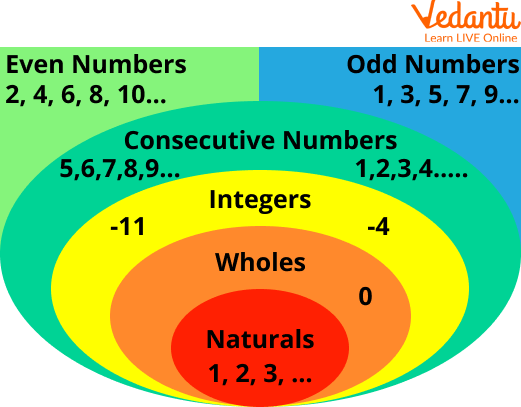Numbers

## Class 3 Maths Chapter 2 Worksheet

Here, we are going to solve questions related to the Class 3 Maths Chapter 2 worksheet.

Q1. oh! 206! How many more are needed to reach a triple century?

Ans: As we know that triple century means 300

This implies we have to make 300 runs.

Thus, 300 – 206 = 94

Implies 94 runs are needed.

Q2. Who am I? Match with the number.

 I come between 40 and 50, and there is a 5 in my name. 96 I have 9 in my name and am very close to 90. 150 If you hit a 4 after me, you score a century. 45 I am equal to ten notes of 10. 89 I am a century + half century 87 I am exactly in between 77 and 97. 100

Ans:

The following are the correct match:

 I come between 40 and 50, and there is a 5 in my name. 45 I have 9 in my name and am very close to 90. 89 If you hit a 4 after me, you score a century. 96 I am equal to ten notes of 10. 100 I am a century + half century 150 I am exactly in between 77 and 97. 87

## Maths Games for Grade 3

There are Maths games for grade 3 that you can play without computers i.e.

• Prodigy maths

• Maths baseball

• Bouncing sums

• Maths is fun

## Solved Examples

Q1. Which of the following is not an even number: 2, 3, 4, 6, 8?

Ans: An even number is a number that is a multiple of 2. It can be said that any number that is completely divisible by 2 is an even number.

Therefore, 3 is the number that can’t be divisible by 2. Hence, 3 is not an even number.

Q2. What is LVI in Roman numerals?

Ans: We know that L = 50, V = 5, and I = 1.

So we will expand and add the values of all the letters.

This will be L + V + I = 50 + 5 + 1 = 56.

Q3. Complete the series i.e. 1, 3, 6, 10, ____

Ans. We have given that 1, 3, 6, 10,?

1 + 2 = 3

3 + 3 = 6

6 + 4 = 10

10 + 5 = 15

Q4. Which of the following numbers is greater, 89 or 98?

Ans: As we have learned while comparing numbers, we always take the highest place value first. Here, at tens place; we can see that 8 < 9. Hence, 89 < 98.

## Practice Questions

Q1. What does XXVI mean in numbers?

Ans: 26

Q2. Write all the even numbers between 155 and 165.

Ans: 156, 158, 160, 162, 164

Q3. Are cylinders and oval both 3D shapes?

Ans: No, only the cylinder comes under a 3D shape.

Q4. 3 friends want a bike and a car. Rahul had Rs.48.50, Rishi had Rs.55.50, and sona had Rs.38.00. find how much money they all have.

Ans: Rs.142

## Summary

4-digit numbers are numbers having 4 digits, and we can form 4-digit numbers by using any digits from 0-9, but the number should begin with a digit 1 or a number greater than 1. Roman numerals are the symbolic representation of numbers that do not follow a place value system. They comprise Latin alphabets I, V, X, L, C, D, and M. These are used to represent the numbers 1, 5, 10, 50, 100, 500, and 1000.

Comparing numbers is a method of comparing two or more numbers and identifying if one number is equal, lesser, or greater than the other numbers. When comparing natural numbers, the number with more digits is greater than the number with a lesser number of digits. Geometric shapes are also known as geometric figures made up of a combination of lines or curves. Then in the end, we added some solved and practice questions, to get a better command of the topic.

Last updated date: 24th Sep 2023
Total views: 90.3k
Views today: 1.90k

## FAQs on Knowledge of Maths for Class 3

1. What is the use of geometric shapes?

Geometric shapes refresh our visual senses in a logical, practical, and interesting way. They give a sense of order and accuracy to a piece of work and everyday life.

2. What is the smallest 4-digit number?

1,000 is the smallest 4-digit number. We can read the number in words as one thousand. The number before 1,000 is 999 and it is a three-digit number, therefore, the smallest 4-digit number is 1,000.

3. Where are Roman numerals used in everyday life?

Roman numerals are used in the French Republican Calendar. They are also used to denote the varying levels of brightness in photography, the magnitude of earthquakes in seismology, page numbering of books, chapter numbers, and so on.